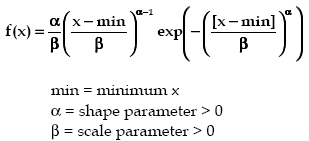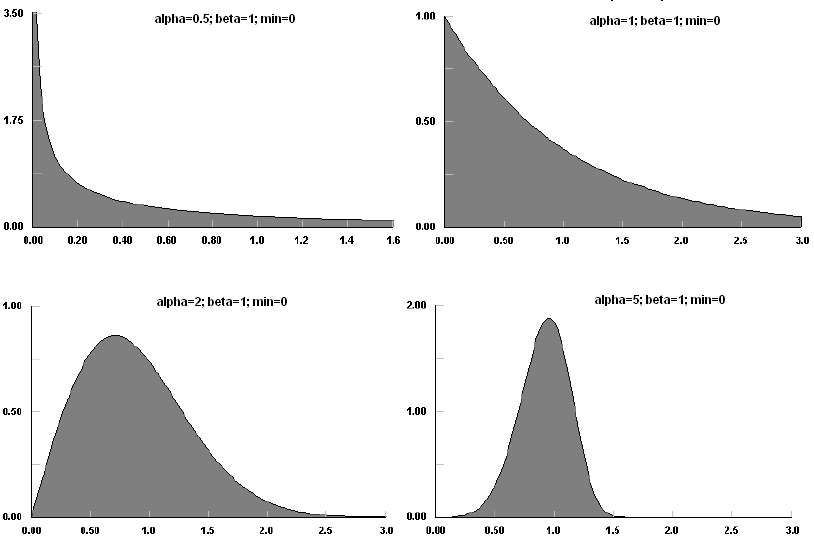AnyLogic

# weibullThe Weibull distribution is a continuous distribution bounded on the lower side. Because it provides one of the limiting distributions for extreme values, it is also referred to as the Frechet distribution and the Weibull-Gnedenko distribution.

Like the Gamma distribution, it has three distinct regions. For alpha=1, beta=1/lambda the Weibull distribution is reduced to exponential (lambda) distribution, starting at a finite value at minimum x and decreasing monotonically thereafter. For alpha < 1, the Weibull distribution tends to infinity at minimum x and decreases monotonically for increasing x. For alpha > 1, the Weibull distribution is 0 at minimum x, peaks at a value that depends on both alpha and beta, decreasing monotonically thereafter. Uniquely, the Weibull distribution has negative skewness for alpha > 3.6.

The Weibull distribution can also be used to approximate the Normal distribution for alpha=3.6, while maintaining its strictly positive values of x [actually (x-min)], although the kurtosis is slightly smaller than 3, the Normal value.

The Weibull distribution derived its popularity from its use to model the strength of materials, and has since been used to model just about everything. In particular, the Weibull distribution is used to represent wearout lifetimes in reliability, wind speed, rainfall intensity, health related issues, germination, duration of industrial stoppages, migratory systems, and thunderstorm data.

### Samples### weibull(double alpha, double beta, double min)

Description
Generates a sample of the Weibull distribution.
Parameters
Name Type of value Description
alpha double the shape parameter > 0
beta double the scale parameter > 0
min double the minimum x value
Result
Type Description
double the generated sample

### weibull(double beta, double alpha)

Description
Generates a sample of the Weibull distribution with min set to 0. Is equivalent to weibull(alpha, beta, 0).
Parameters
Name Type of value Description
beta double the scale parameter > 0
alpha double the shape parameter > 0
Result
Type Description
double the generated sample

### weibull(double alpha, double beta, double min, java.util.Random r)

Description
Generates a sample of the Weibull distribution using the specified random number generator.
Parameters
Name Type of value Description
alpha double the shape parameter > 0
beta double the scale parameter > 0
min double the minimum x value
r java.util.Random the random number generator
Result
Type Description
double the generated sample

This document includes content from the Stat::Fit User's Manual. Copyright 2016 Geer Mountain Software Corp.